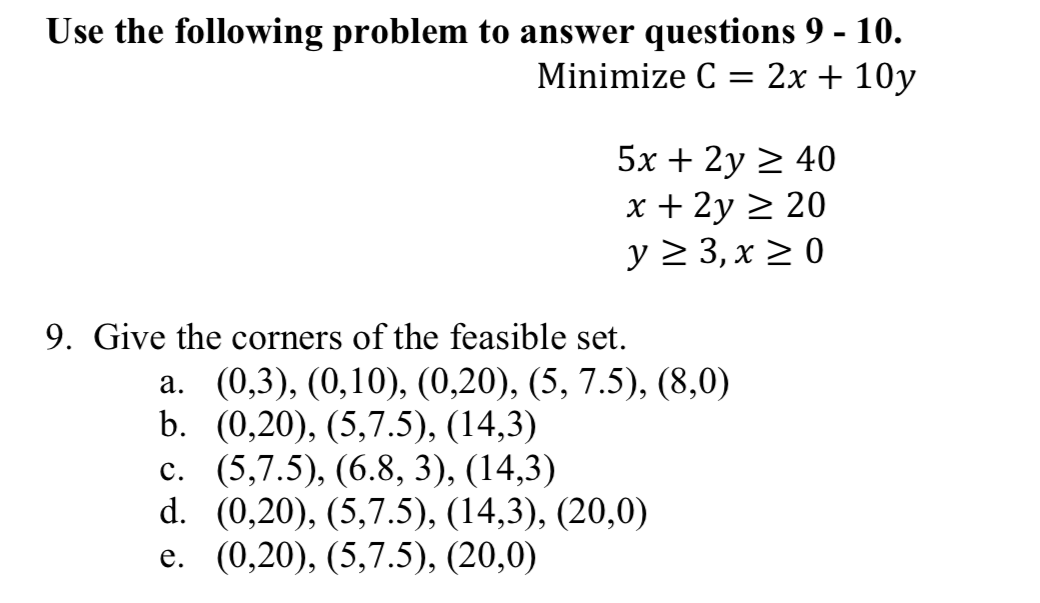# Use the following problem to answer questions 9 - 10.Minimize C-2x + 10y5x 2y 2x + 2y40209. Give the corners of the feasible set.a. (0,3), (0,10), (0,20), (5, 7.5), (8,0)b. (0,20), (5,7.5), (14,3)c. (5,7.5), (6.8, 3), (14,3)d. (0,20), (5,7.5), (14,3), (20,0)e. (0,20), (5,7.5), (20,0)

Question
10 viewshelp_outlineImage TranscriptioncloseUse the following problem to answer questions 9 - 10. Minimize C-2x + 10y 5x 2y 2 x + 2y 40 20 9. Give the corners of the feasible set. a. (0,3), (0,10), (0,20), (5, 7.5), (8,0) b. (0,20), (5,7.5), (14,3) c. (5,7.5), (6.8, 3), (14,3) d. (0,20), (5,7.5), (14,3), (20,0) e. (0,20), (5,7.5), (20,0) fullscreen
check_circle

Step 1

We have given, constraint equations and a function that needs to be minimized.

Step 2

We can draw constraint equations...

### Want to see the full answer?

See Solution

#### Want to see this answer and more?

Solutions are written by subject experts who are available 24/7. Questions are typically answered within 1 hour.*

See Solution
*Response times may vary by subject and question.
Tagged in

### Linear Programming